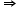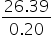Maths-
General
Easy

Question

# The Price of Stock A at 9 A.M was 12.73 .Since then the price has been increasing at the rate of Rs 0.06 per hour. At noon , the price of stock B was Rs 13.48 .It begins to decrease at the rate of Rs 0.14 per hour. If the stocks continue to increase and decrease at the same rates, in how many hours will the prices of the stocks be the same?Hint:

## The correct answer is: ⇒ x = 131.95 hours

### ○ Given:Stock 1:  price of stock at 9 A.M =  Rs. 12.73Rate of increase = Rs. 0.06 per hourStock 2:  price of stock at 12 P.M =  Rs. 13.48Rate of decrease = Rs. 0.14 per hour.○ Step 1:○ First calculate price of stock at 12 P.MPrice of Stock 1 at 12 P.M will be12.73 + (0.06  3)  {multiply by three as 3 hours will pass till 12 P.M from 9 A.M}12.73 + 0.18 = 12.91So, the price of Stock 1 at 12 P.M will be Rs. 12.91○ Step 2:○ Let x hours after 12 P.M their price will be sameFirst, consider case of stock 1:Price of Stock 1 after x hours from 12 P.M will be.12.91 + 0.06xNow, consider case of Stock 2:Price of Stock 2 x hours after 12 P.M will be13.48 - 0.14x   { subtraction as it is decreasing }○ Step 3:○ Now equate the price of stock x hours after 12 P.M:12.91 + 0.06x = 13.48 - 0.14x0.06x + 0.14x = 13.48 - 12.910.20x = 26.39x =x = 131.95 hours Final Answer: Hence, after 131.95 hours value of stock will be equal.

The equation is defined as a mathematical statement with at least two terms containing variables or numbers that are equal.
Let's take an example:
Assume the hours are "h" when attempting to equalize,
As per the given question, we can write the equation as:
12.73 + 0.06h = 13.48 - 0.14h
Rearrange the terms of h in the above equation,
0.06h + 0.14h = 13.48- 12.73
In the above equation, combine the corresponding terms,
0.2h = 0.75
Divide both sides by 0.2
h = 0.75/0.2
h = 3.75
Thus, the stock prices will be the same in 3.75 hours.#### With Turito Foundation.#### Get an Expert Advice From Turito.